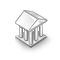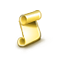Interesting script?
So post a link to it -
let others appraise it

You liked the script? Try it in the MetaTrader 5 terminalMath Utils (MT4) - library for MetaTrader 4

Views:
452
Rating:
Published:
2019.06.04 20:18
Updated:
2019.06.04 21:19

Handy functions for comparison of floating-point numbers

```bool EQ(double a, double b, int digits = 8);

bool GT(double a, double b, int digits = 8);

bool LT(double a, double b, int digits = 8);

bool NE(double a, double b, int digits = 8);

bool GE(double a, double b, int digits = 8);

bool LE(double a, double b, int digits = 8);```

Handy functions for rounding of floating-point numbers

```double RoundToDigit(double number, int digits);

double RoundToDigitUp(double number, int digits);

double RoundToDigitDown(double number, int digits);

double RoundToStep(double number, double step);

double RoundToStepUp(double number, double step);

double RoundToStepDown(double number, double step);

double RoundPrice(double pPrice, string pSymbol = NULL);

double RoundVolume(double pVolume, string pSymbol = NULL);```

Exact comparison of doubles

Sometimes, when comparing two double numbers that assumed to be equal, but reached to via different calculations, the comparison goes wrong. Actually A can differ from B by in very little amount (at the 16th decimal place) due to binary rounding errors, so exact comparisons (==!=>>=<<= operators) fail.

Real situations of where exact comparison of doubles can fail

• Comparing doubles reached via different calculations:

```if (0.1+0.2 == 0.3)  // false
```
• Experts with trailing stop function:

```if (new_sl > OrderStopLoss())
if (new_sl < OrderStopLoss())```
• Experts with hidden take profit function:

```if (Bid >= HiddenTakeProfit)
• Comparing price levels in a grid strategy:

```if (Ask < virtual_stopLoss)
if (Bid > virtual_stopLoss)```

Loose Comparison

To avoid unexpected results, it is better to replace exact comparisons (==!=>>=<<=), with loose comparisons to overcome the floating-point imprecision. This small library provides the required functions.

```//--- exact comparisons can fail
if (Bid > virtual_stopLoss)
if (0.1+0.2 == 0.3)  // false

//--- using library functions, results become as expected
if (GT(Bid, virtual_stopLoss))
if (EQ(0.1+0.2, 0.3))  // true
```indicator for day trading 5 min chart

This is useful if you want to earn and work on 5 min chart . it uses bb , ma 10, ma50 , Psar, slow s, one faster s and macd along with rsi to give a perfect view of market . if you need some help let me know in my email. rinkanrohitjena@live.com only works in mq4 language . just drag and drop on a five min chartJohnBollinger_Ovrl

EA based on parameters of the BOOK of John Bollinger with Lot size calculator and position overlaping.Adaptive Moving Average - AMACrypto S&R